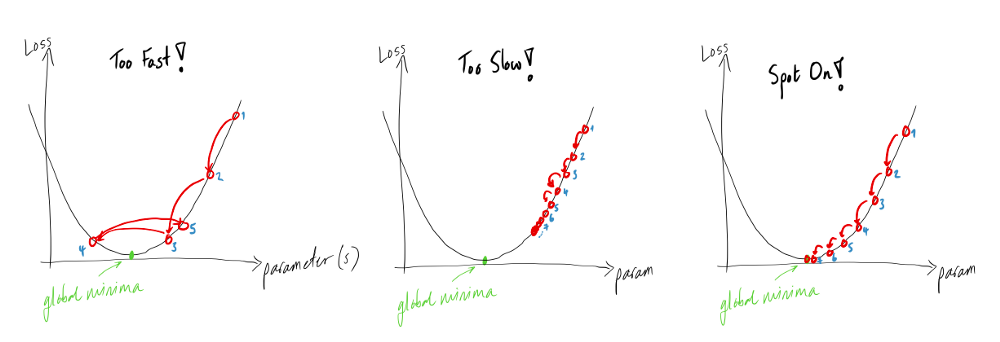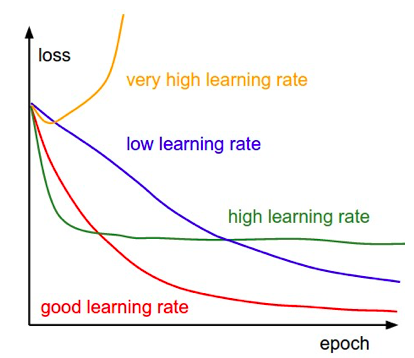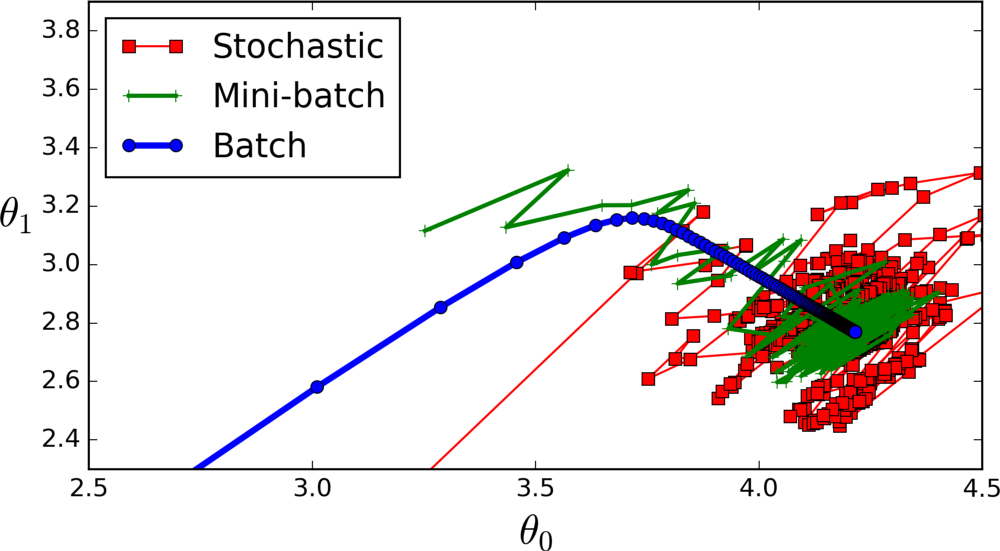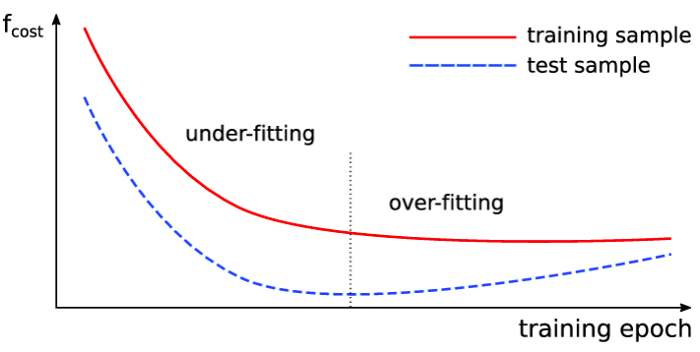## Hyperparameter Tuning

Posted by Mayur

### What is Hyperparameter

Hyperparameter is a static parameter, which needs to be assigned a value before applying an algorithm to data. For instance, parameters like learning rate, epochs, etc, are set before training the models.

Optimization Hyperparameter- These parameters are related to optimization processes like gradient descent (learning rate), training process, mini-batch sizes, etc.

Model Hyperparameter- These parameters are related to models like several hidden layers or number of neurons in each layer etc.

### Learning Rate

It is the most important of all hyperparameters. Even for a pre-trained model, we should try out multiple values of the learning rate. The most commonly used learning rate is 0.1, 0.01, 0.001, 0.0001, 0.00001 etc.Figure 1: Learning Rate

A large value of learning rate tends to overshoot the gradient value making it difficult for the weight to converge to the global minimum.

A small value of learning rate makes the convergence towards the global minimum very slow. We can recognize this from the training and validation loss.

An optimum value of learning rate will lead to a global minimum, which can be viewed by constantly decreasing loss.

### Learning Rate Decay

Keeping only one learning rate may not help the weight to reach the global minimum. So we can change the value of the learning rate after a certain number of epochs. It helps gradient stuck in a local minimum.Figure 2: Learning Rate Decay

Sometimes it is crucial to understand the problem and change the learning rate accordingly, like increasing or decreasing it. Functions like Adam and Adagrad Optimizer helps in adapting the learning rate following the objective function.

### Minibatch Size

It is one of the most commonly tuned hyperparameters in deep learning. Let’s consider we have 1000 records and we have to train a model on top of it. Now, for training, we can select different batch sizes for the model. Let’s check out different batch sizes.

First

If we keep Minibatch size = 1, then the weights are updated for every record after backpropagation. It is called Stochastic Batch Gradient Descent.Figure 3: Minibatch

Second

If the Minibatch Size = # of records in the dataset, then the weight update is done after all the records are passed through the forward propagation. It is called Batch gradient descent.

Third

If the Minibatch Size = value between 1 to total no. of records, then the weight update is done after the set values of records are passed through the forward propagation. It is called Mini-batch gradient descent.

The most commonly used value for Minibatch sizes is 32, 64, 128, 256. Values more than 256 require more memory and computational efficiency.

### Number of Epochs

The number of epochs is decided based on the validation error. As the validation error keeps reducing, we can assume that our model is learning and updating the weights positively.

There is also a technique called early stopping, which helps in determining the no. of iterations.Figure 4: Iterations

Validation Monitor


validation_monitor = tf.contrib.learn.monitors.ValidationMonitor(
test_set.data,
test_set.target,
every_n_steps=50,
metrics=validation_metrics,
early_stopping_metric="loss",
early_stopping_metric_minimize=True,
early_stopping_rounds=200)



The last parameter indicates the ValidationMonitor. It suggests that the training process should stop if the loss doesn’t decrease in the 200 training steps (rounds).

StopAtStepHook

A monitor to request the training to stop after a certain number of steps.

NanTensorHook

It monitors losses and stops training if it encounters a NaN loss.

### Number of Hidden Units/Layers

Highly mysterious hyper-parameters to decide is the number of hidden units and layers. The objective of the deep learning model is to build a complex mapping function between features and targets.

In complex mapping, the complexity is directly proportional to the number of hidden units. More number of hidden units leads to more complex mapping.

Note, if we create too complex a model, then it overfits the training data. We can see this from the validation error while training, then in such a case, we should reduce the hidden units in that case.

To conclude, keep track of validation errors while increasing the number of hidden units.

*As stated by Andrej Karpathy, a three-layer net outperforms the two-layer net but going beyond that rarely helps the network. While in CNN, the more the number of layers, the better is the performance.

If you’ve liked this post, please don’t forget to subscribe to the newsletter.